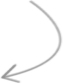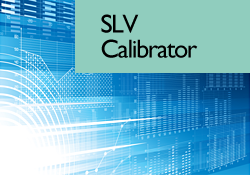Stochastic Local Volatility Calibrator | SciComp Inc.

# Stochastic Local Volatility Calibrator

The Stochastic Local Volatility Calibrator is based on the work of Ren, Madan and Qian [Risk, Sept 2007], Lipton [Risk, Feb 2002], Jex, Henderson and Wang [J.P.Morgan, 1999], and the Bloomberg paper "Stochastic Local Volatility" by Tataru & Fisher.

The Stochastic Local Volatility Calibrator takes any calibrated LV surface that matches vanillas. Then, using a non-linear Fokker-Planck equation, one adds a SV component and for any given set of SV parameters computes a new "leveraged local volatility surface" that still matches the vanillas, while accommodating SV. The Stochastic Local Volatility Calibrator then applies to this PDE solution a Levenberg-Marquardt optimizer and finds the (time bucketed) SV parameters that yield a maximally flat leveraged local volatility surface.

At this point you have the pure LV model (the original LV surface) and the pure SV model (the SV parameters that yield a nearly flat leveraged LV surface). Both models fit the vanillas. The Stochastic Local Volatility Calibrator then solves for a mixing fraction then mixes the two models together either by a) calibrating to selected exotics, or b) using historical data.

### Test Drive the Stochastic Local Volatility Calibrator.